•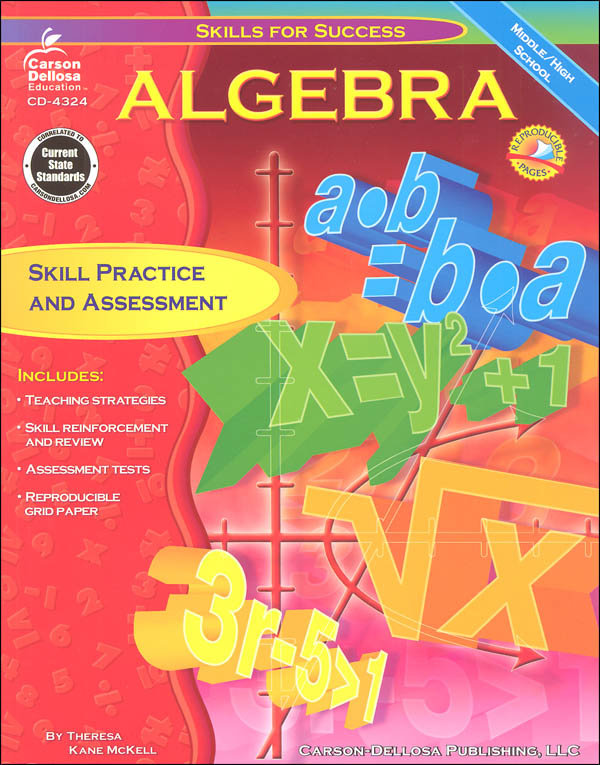•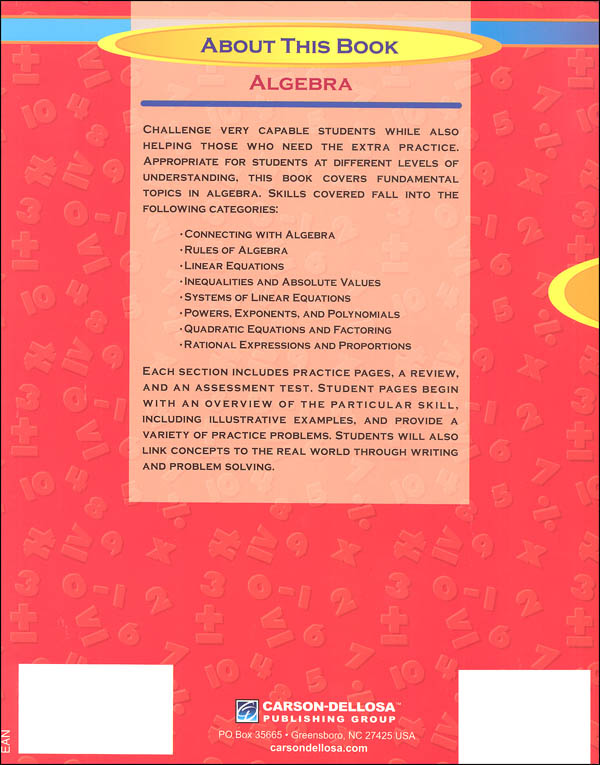•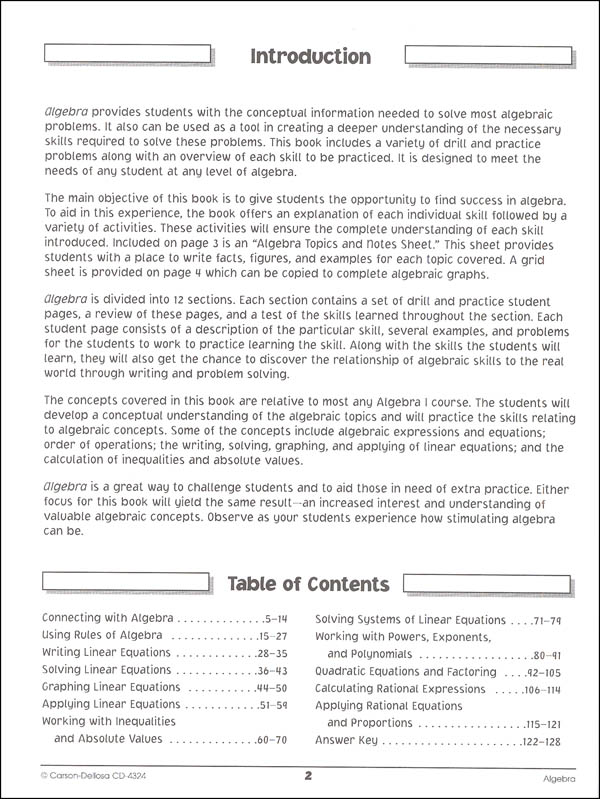•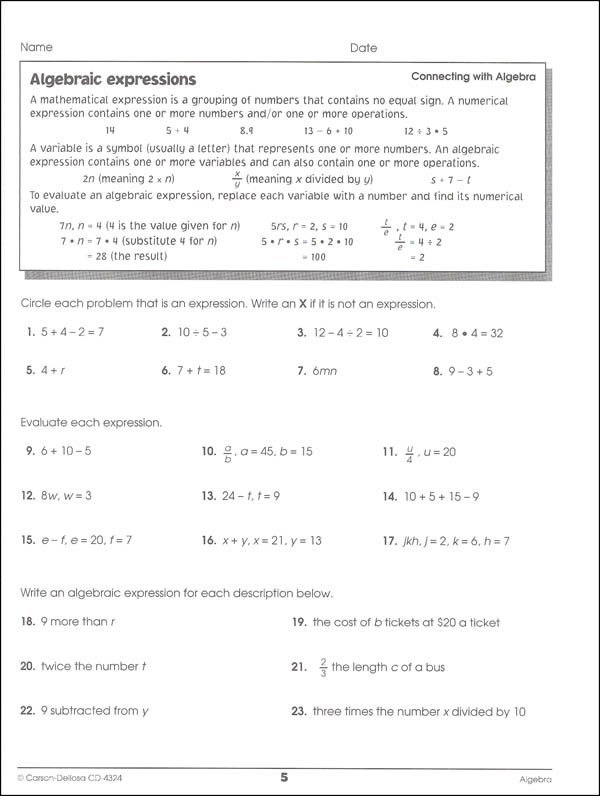•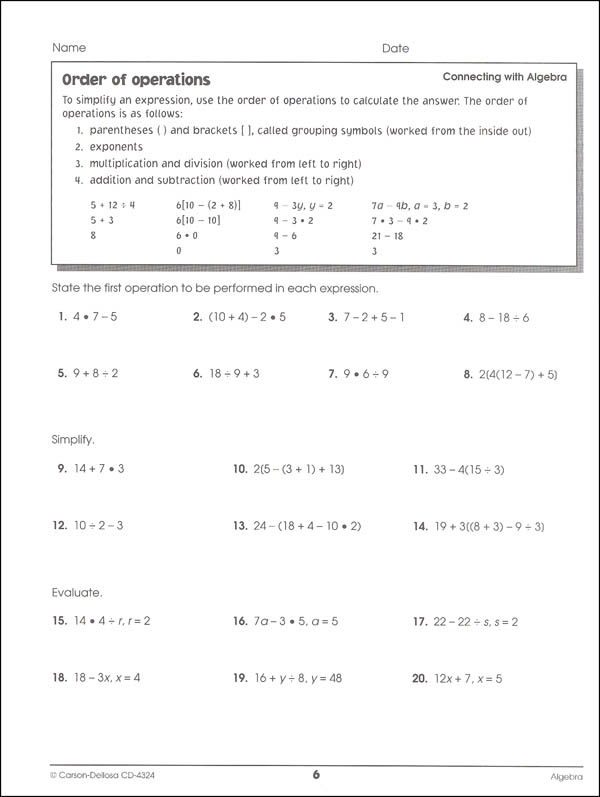# Algebra (Skills for Success)

# 034647

Our Price: \$11.95
Retail: \$16.99
Save: 29.66% (\$5.04)
In Stock.
Qty:
Qty:

Item #: 034647 9780887249358 6-12

#### Product Description:

Add some extra practice and review to your curriculum with the Skills for Success Algebra workbook. The book is divided into twelve sections connecting with algebra, using rules of algebra, writing linear equations, solving linear equations, graphing linear equations, applying linear equations, working with inequalities and absolute values, solving systems of linear equations, working with powers/exponents/polynomials, quadratic equations and factoring, calculating rational expressions, and applying rational equations and proportions. The topical organization make it easier to match the content with your curriculum. Each section includes practice pages, review, and a test; the number of pages varies by section. There are 128 pages and are reproducible for classroom and family use and answer keys are included. A little extra practice never hurts! ~ Donna

#### Publisher Description:

Perfect for students at different levels of understanding, Algebra for middle school and high school students provides teaching strategies as well as skill reinforcement and review.

Topics in this 128-page math resource book include these fundamental algebra skills:

• linear equations
• inequalities and absolute values
• systems of linear equations
• powers
• exponents
• rational expressions

This comprehensive algebra practice book supports NCTM standards and also includes practice pages, assessment tests, reproducible grid paper, and an answer key.

Features:
Primary Subject
Mathematics
6
12
ISBN
9780887249358
Format
Paperback
Brand Name
Carson-Dellosa
Weight
0.8 (lbs.)
Dimensions
10.81" x 8.5" x 0.25"